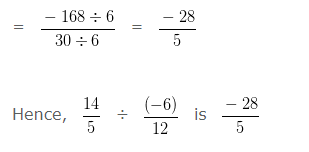# Rational Numbers Class 7 Questions

Rational Numbers Class 7 Questions, deals with various concepts which are as under:-

• Additive Inverse of a Rational Number
• Equivalent Rational Numbers
• Subtracting Rational Numbers
• Multiplication of Rational Numbers
• Dividing Rational Numbers

#### Rational Numbers Class 7 Questions – Equivalent Rational Numbers

• To obtain a rational number equivalent to the given rational number we have to multiply the numerator and denominator of a given rational number by the same nonzero number.
• To obtain a rational number equivalent to the given rational number we have to divide the numerator and denominator of a given rational number by a common divisor.

Example 1

Are 3/5 and 9/15 are equivalent Rational Numbers?

Explanation:

If two Rational Numbers are equivalent, the product obtained by Cross Multiplying them would also be equal

On Cross Multiplying the given Rational numbers

3/5 and 9/15

we get,

3 x 15 = 45

and 9 x 5 = 45

Since, 3 x 15 = 9 x 5

So, 3/5 = 9/15

Hence, 3/5 and 9/15 are equivalent Rational Number.

Equivalent Rational Number with given Numerator

Example 2

Express -8/6 as a Rational Number with Numerator 24.

Explanation:

Numerator of -8/6 = -8

We need to change the Numerator of -8/6 to 24

We need to find a number, with which we should multiply -8 so it is equal to 24

To obtain that number, we would need to divide 24 by -8 i.e,

24/(-8) = -3

So, we have to multiply both the Numerator and Denominator of given Rational Number by -3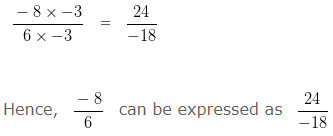Equivalent Rational Number with given Denominator

Example 3

Express 16/25 as a Rational Number with Denominator -75.

Explanation:

Denominator of 16/25 = 25

We need to change the Denominator of 16/25 to -75

We need to find a number, with which we should multiply 25 so it is equal to -75

To obtain that number, we would need to divide -75 by 25 i.e,

(-75)/25 = -3

So, we have to multiply both the Numerator and Denominator of given Rational Number by -3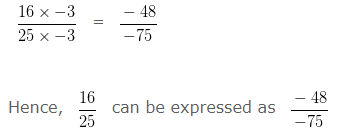#### Rational Numbers Class 7 Questions – Additive Inverse of a Rational Number

Additive inverse of any rational number is that number with minus (negative) sign before it.
i.e, Additive inverse of a/b is -a/b

Example 4

Explanation:

Additive inverse of any number is that number with minus (negative) sign before it.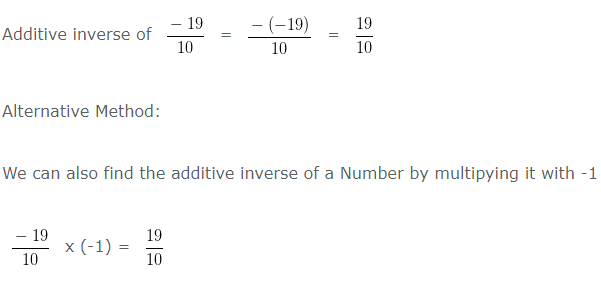When we have to add two rational numbers, First we should convert each of them into a rational number with a positive denominator.

Addition of Rational Numbers When Denominator Are Equal

Question 5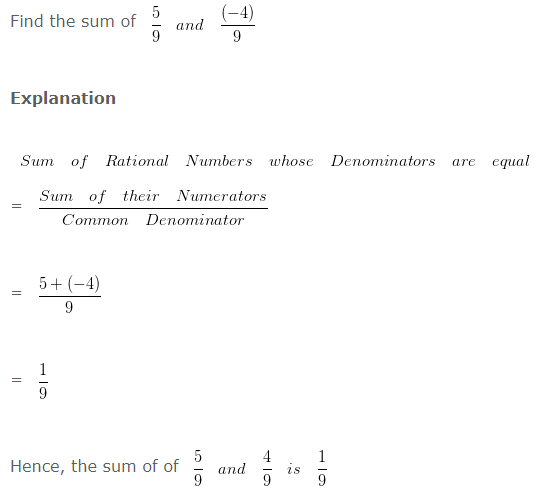Question 6

Find the sum of 19/13 and 11/(-13)

Explanation

To add two Rational Numbers, first their Denominators should be positive

So, we would first express 11/(-13) as a Rational Number with a positive Denominator.

Multiplying both the Numerator and Denominator by (-1)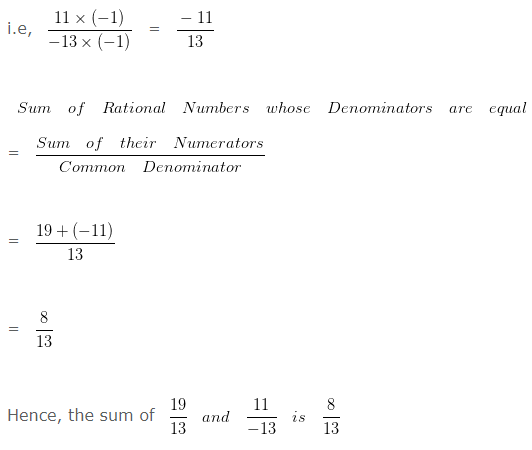Addition of Rational Numbers When Denominators Are Unequal

Question 7

Find the sum of 7/6 and 5/9?

Explanation

To add two Rational Numbers with different Denominator, we will first find the LCM of both the Denominators.

LCM of 6 and 9 is 18

Now we would divide such LCM by Denominator of first number and the result would be multiplied with both the numerator and denominator of such number

Dividing LCM by the Denominator of first number

18 ÷ 6 = 3

Multiplying both the Numerator and Denominator of 7/6 by the quotient i.e, 3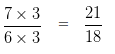Dividing LCM by the Denominator of Second number

18 ÷ 9 = 2

Multiplying both the Numerator and Denominator of 5/9 by 2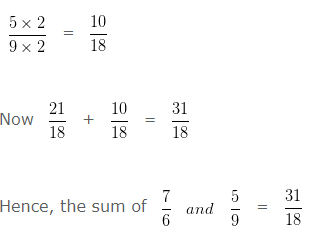Question 8

Find the sum of 9/8 and 5/(-6)?

Explanation

first

their Denominators should be positive

So, we would first express 5/(-6) as a Rational Number with positive Denominator.

Multiplying both the Numerator and Denominator by (-1)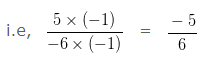To add two Rational Numbers with different Denominator first we will find the LCM of both the Denominators.

LCM of 8 and 6 is 24

Divide LCM by the Denominator of first number

24 ÷ 8 = 3

We have to multiply , both the Numerator and Denominator of 9/8 by the quotient i.e, 3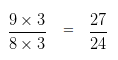Divide LCM by the Denominator of second number

24 ÷ 6 = 4

We have to multiply , both the Numerator and Denominator of -5/6 by 4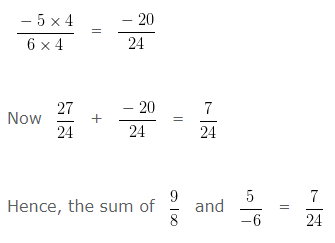#### Rational Numbers Class 7 Questions – Subtracting Rational Numbers

Subtracting Rational Numbers When Denominators are Equal

Question 9

Subtract:

13/7 – 8/7

Explanation

Difference of Rational Numbers when Denominators are equal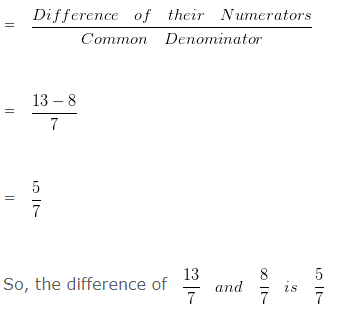Question 10

Subtract:

34/13 – 12/13

Explanation

Difference of Rational Numbers when Denominators are equal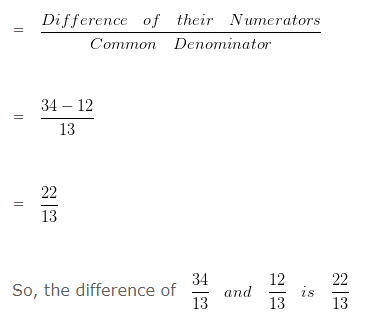Subtracting Rational Numbers When Denominators are Unequal

Question 11

Subtract:

3/4 – 2/7

Explanation

To subtract two Rational Numbers with different Denominators, first we will find the LCM of both the Denominators.

LCM of 4 and 7 is 28

Now we would divide such LCM by Denominator of first number and the result would be multiplied with both the numerator and denominator of such number

Divide LCM by the Denominator of first number

28 ÷ 4 = 7

We have to multiply , both the Numerator and Denominator of 3/4 by the quotient i.e, 7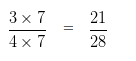Divide LCM by the Denominator of second number

28 ÷ 7 = 4

We have to multiply , both the Numerator and Denominator of 2/7 by the quotient i.e, 4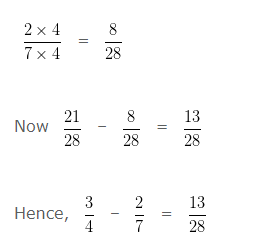Question 12

Subtract:

5/4 – 3/5

Explanation

To subtract two Rational Numbers with different Denominators, first we will find the LCM of both the Denominators.

LCM of 4 and 5 is 20

Now we would divide such LCM by Denominator of first number and the result would be multiplied with both the numerator and denominator of such number

Divide LCM by the Denominator of first number

20 ÷ 4 = 5

We have to multiply , both the Numerator and Denominator of 5/4 by the quotient i.e, 5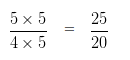Divide LCM by the Denominator of second number

20 ÷ 5 = 4

We have to multiply , both the Numerator and Denominator of 3/5 by the quotient i.e, 4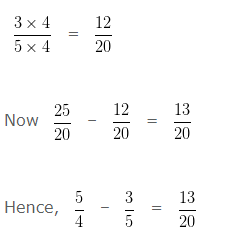#### Rational Numbers Class 7 Questions – Multiplication of Rational Numbers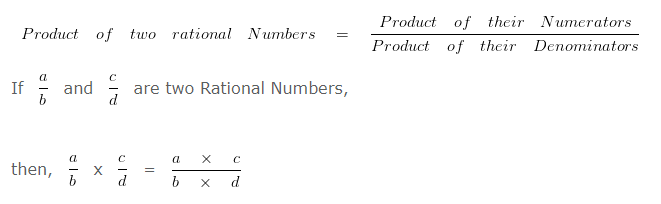Question 13

Find the product of 15/7 and 3/5?

Explanation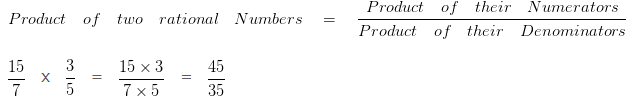To further simplify the given numbers into their lowest form, we would divide both the Numerator and Denominator by their HCF

HCF of 45 and 35 is 5

Dividing both the Numerator and Denominator by their HCF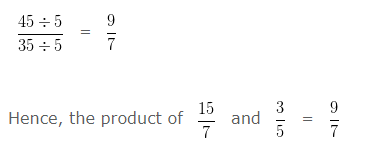Question 14

Find the product of 9/7 and (-12)/8

Explanation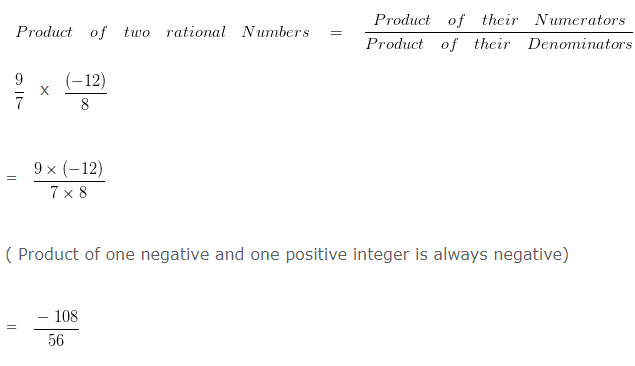To further simplify the given numbers into their lowest form, we would divide both the Numerator and Denominator by their HCF

HCF of 108 and 56 is 4

Dividing both the Numerator and Denominator by their HCF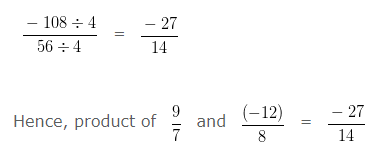Question 15

Find the product of (-4)/7 and 8/12?

Explanation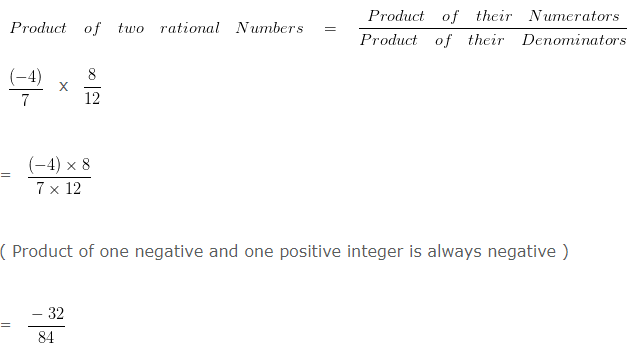To further simplify the given numbers into their lowest form, we would divide both the Numerator and Denominator by their HCF

HCF of 32 and 84 is 4

Dividing both the Numerator and Denominator by their HCF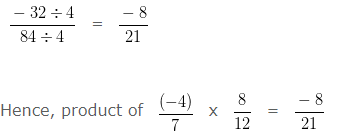Question 16

Find the product of (-11)/8 x (-6)/5?

Explanation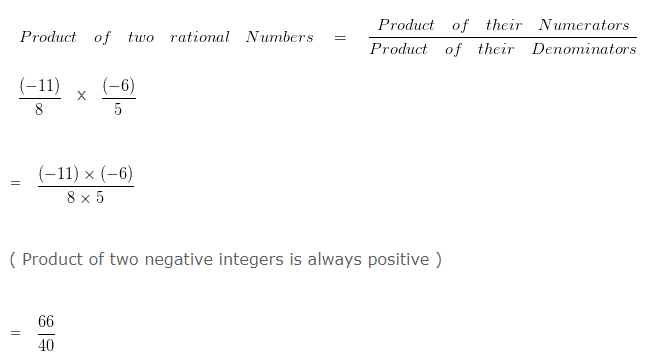To further simplify the given numbers into their lowest form, we would divide both the Numerator and Denominator by their HCF

HCF of 66 and 40 is 2

Dividing both the Numerator and Denominator by their HCF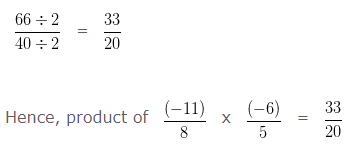#### Rational Numbers Class 7 Questions – Dividing Rational Numbers

In order to divide a Rational Number by another Rational Number
We have to multiply first Rational Number with Reciprocal of the second Rational Number.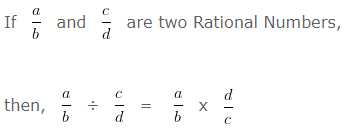Question 17

Divide:

9/7 ÷ 3/4

Explanation

We have, 9/7 ÷ 3/4

In order to divide a Rational Number by another Rational Number

We have to multiply first Rational Number with Reciprocal of the second Rational Number.

We have 9/7 ÷ 3/4

(Reciprocal of 3/4 is 4/3 )

So we can say that,

9/7 ÷ 3/4

= 9/7 x 4/3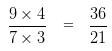To further simplify the given numbers into their lowest form, we would divide both the Numerator and Denominator by their HCF

HCF of 36 and 21 is 3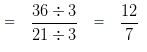Question 18

Divide:

14/5 ÷ (-6)/12

Explanation

We have, 14/5 ÷ (-6)/12

In order to divide a Rational Number by another Rational Number

We have to multiply first Rational Number with Reciprocal of the second Rational Number

Since, Reciprocal of (-6)/12 is 12/(-6)

We can write the given equation as 14/5 ÷ (-6)/12

= 14/5 x 12/(-6)

To make the Denominator positive, we would multiply 12 and -6 by -1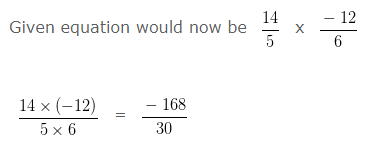To further simplify the given numbers into their lowest form, we would divide both the Numerator and Denominator by their HCF

HCF of 168 and 30 is 6